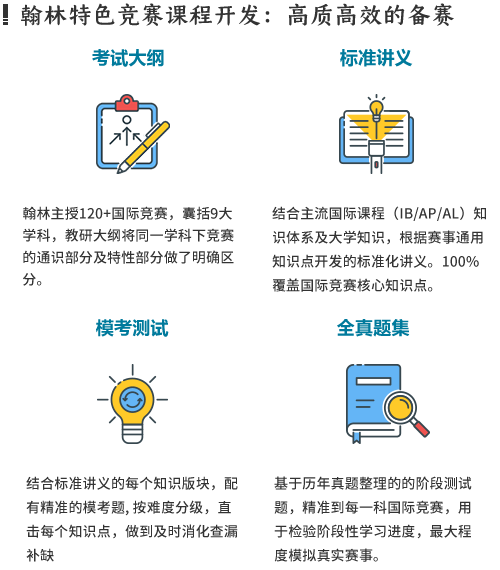# 2022SASMO全程班

3-8人小班，满3人开班

## 描述### 课程须知

3-8人小班辅导：小组教学，针对辅导，及时反馈，性价比高

### 课程大纲

Grade 2-4 (Primary 2-4)
Arithmetic and Statistics
Geometry and Mensuration
Solving word problems using model method (or any other non-algebraic
methods)
Non-routine problem solving (including number patterns, divisibility tests,
spatial visualisation, logic problems and simple cryptarithms)
Grade 5-6 (Primary 5-6)
Arithmetic and Statistics
Geometry and Mensuration
Solving word problems using model method (or any other methods
including algebra)
Non-routine problem solving (including number patterns, divisibility tests,
spatial visualisation, logic problems and cryptarithms)
Grade 7 (Secondary 1)
Arithmetic and Algebra
Geometry, Graphs and Mensuration
Statistics
Non-routine problem solving (including number patterns, divisibility tests,
spatial visualisation, logic problems and cryptarithms)
Grade 8 (Secondary 2)
Arithmetic and Algebra
Geometry, Graphs and MensurationPythagoras’ Theorem
Statistics
Non-routine problem solving (including number patterns, divisibility tests,
spatial visualisation, logic problems and cryptarithms)
Grade 9-10 (Secondary 3-4)
Arithmetic and Algebra
Geometry, Graphs and Mensuration
Pythagoras’ Theorem and Trigonometry
Statistics and Probability
Non-routine problem solving (including number patterns, divisibility tests,
spatial visualisation, logic problems and cryptarithms)

### 授课老师

5年美国生活、学习、工作经验。研究生期间担任大学助教，带教的多名学生获得学院奖学金，并有学生顺利拿到名校研究生Offer。